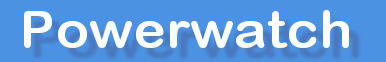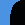» Printer friendly version

# Conversion of RF units (V/m » W/m2 and vice versa)

Science index » Overview | Article library | List of studies | Basic guide to EMFs | International guidance levels | Unit conversion | Frequently asked questions | Other resources

Many people ask us for conversions between microwave measurement units so that they can compare the units we use, volts per metre (V/m), with watts per metre squared (W/m2). For modern digital telecommunications signals, this is not as easy, or as useful, as you would first think that it would be.

For continuous-wave transmissions, including VHF FM radio signals, the conversion is relatively easy. These signals remain fairly constant in amplitude and the conversion from signal strength in volts/metre to power flux density (PFD) in watts per square metre can be done using the formula:

PFD = (V/m)2/377 watts per metre squared (W/m2)

e.g. 58.2 V/m (ICNIRP 1800 MHz) = (58.2*58.2)/377 = 9 W/m2

This conversion is not particularly relevant for exposure from mobile phones, base stations and DECT cordless phones and the results can be extremely misleading.

The problem occurs because PFD is ONLY relevant to heating and it averages the power over time (6 minutes for official RF PFD measurements). Any PFD has to be integrated over time and most hand-held instruments average over at least a few seconds. Some instruments have a "peak-detect" facility that can give the equivalent power as if the pulsing peak levels were continuous. Note this is not the same as a "max hold" facility. This is because the normal max hold function on a meter gives you the maximum RMS value measured over the time you have been using the instrument, whereas peak detect measures the level at the top of any pulses in the signal.

For example, the peak power from a TETRA base station is twice the average power. The peak power of a DECT cordless phone base unit can be up to 100 times more than the average power

Powerwatch believes that the best unit of measurement for varying microwave signals at the non-thermal levels we are concerned with is volts per metre. Most instruments that display PFD units have actually measured the signal in terms of V/m and then internally calculated the equivalent (usually average) PFD value in W/m2 or similar.

## RF unit converter (signal strength and power flux density (PFD) for continuous (CW) signals)

Make sure you select the correct unit for power flux density before running the conversion:

 Signal strength: V/m Power flux density (PFD): µW/m2 W/m2 mW/cm2